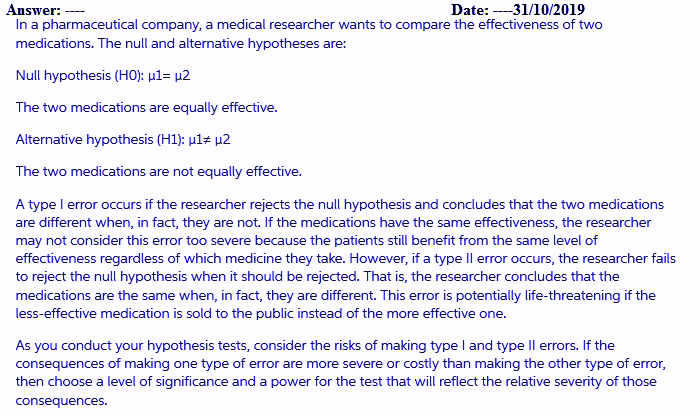In: Math

# When applying statistical tests involving comparing two means or a sample to a population mean, there...

When applying statistical tests involving comparing two means or a sample to a population mean, there are many organizational applications. For example, Human Resources may want to track entrance exam scores of their new hires. This would be an example of two mean comparison. In terms of the recent election, Gallup may take a sample and compare to a population of candidate votes (sample mean compared to a population mean).

Think of an example in your organization of either one of these tests and discuss its application as well as the risk of type 1 or 2 errors.

## Solutions

##### Expert Solution## Related Solutions

##### What is the major advantage to constructing a paired difference experiment when comparing two population means?...
What is the major advantage to constructing a paired difference experiment when comparing two population means? a) The paired difference experiment accounts for dependence between data pairs. b) The paired difference experiment benefits from the fact that selecting samples independently is always optimal c) The test statistic is easier to calculate d)The paired difference experiment guarantees a strong linear correlation
##### Comparing Two Population Means with Known Standard Deviations In this problem you do know the population...
Comparing Two Population Means with Known Standard Deviations In this problem you do know the population standard deviation of these independent normally distributed populations The mean for the first set ¯xx¯ 1 = 15.49 with a standard deviation of σσ 1 = 2.5 There sample size was 13. The mean for the first set ¯xx¯  2  = 15.796 with a standard deviation of σσ 2 = 1.4 There sample size was 18. Find the test statistic z=   Round to 4 places. Find the...
##### Question 1 Tests comparing 2 population means can be: surveys experiments both surveys and experiments Question...
Question 1 Tests comparing 2 population means can be: surveys experiments both surveys and experiments Question 2 If all the experimental units are identical (ex: clones), you will set up the study as: CRD Block Design Question 3 a 2 sample t-test still has which normality assumption(s)? The population is normal or n is greater than 30 both populations are normal or both sample sizes are greater than 30 Question 4 When you block by some variable in a block...
##### Compute the confidence interval for the difference of two population means. Show your work. Sample Mean...
Compute the confidence interval for the difference of two population means. Show your work. Sample Mean 1= 17 Population standard deviation 1= 15 n1= 144 Sample Mean 2= 26 Population Standard Deviation 2= 13 n2 = 121 Confidence Level= 99
##### Comparing two population means and not knowing the population standard deviation I would use which distribution?...
Comparing two population means and not knowing the population standard deviation I would use which distribution? A. Two Sample Z statistic, equation 11-2 B. Two Sample t statistic, equation 11-4, assuming equal variances C. Two Sample t statistic, equation 11-5, assuming unequal variances D. either B or C
##### How are paired t-tests different from two-sample t-tests? 1. When are they used: population parameters 2....
How are paired t-tests different from two-sample t-tests? 1. When are they used: population parameters 2. Data types they are used on 3. Which sample statistics are used as estimates for the population parameter 4. Test statistics, including conditions and Minitab command
##### The distribution of sample means has the same mean as the underlying population, but the standard...
The distribution of sample means has the same mean as the underlying population, but the standard deviation differs. Use a real world scenario to explain why it makes sense the variation decreases as the sample size increases. Please make copy paste available
##### The distribution of sample means has the same mean as the underlying population, but the standard...
The distribution of sample means has the same mean as the underlying population, but the standard deviation differs. Use a real world scenario to explain why it makes sense the variation decreases as the sample size increases. Please make copy paste available
##### A hypothesis will test that two population means are equal. A sample of 10 with a...
A hypothesis will test that two population means are equal. A sample of 10 with a standard deviation of 3 is selected from the first population and a sample of 15 with a standard deviation of 8 from the second population. The standard deviations are not equal. Testing the claim at the 0.01 level, what is the critical value? Assume unequal standard deviations. Please explain and show your work. Thank you!
##### 1.For single-sample statistical tests, if the population variance is unknown you should conduct a (ANSWER) test....
1.For single-sample statistical tests, if the population variance is unknown you should conduct a (ANSWER) test. 2.The critical value for a two-tailed one sample z- test is +/- 3.If the z–score for a sample mean is 1.75, and it was a two-tailed z-test, we (ANSWER) the null hypothesis. 4.A researcher reports the results of a single-sample t-test as t(29) = 1.80. The numerical degrees of freedom for this t-test are (ANSWER), which means there were (ANSWER) total participants in the...